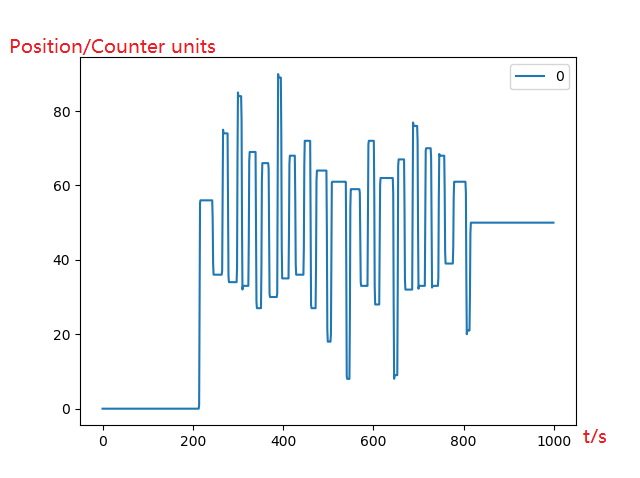Unsatisfied Position Control Performance

Hi ODrive Community,

I am testing the position control feature of ODrive by U8 Lite Tmotor (http://store-en.tmotor.com/goods.php?id=462). After following the instructions on the ODrive website, my motor can run without error, but the performance is still unsatisfied especially the position control. The problems are:

1, The motor always fails to maintain a speed lower than 80 r/min. It repeats the procedure of startup to a relatively higher speed - speed going down towards desired low speed - motor stops.

2, The ODrive takes a long time to control the motor to rotate to the desired position. It fluctuates around the desired point for many and unpredictable times until it finally reach as shown in the pic below.I guess the solution is to precisely tuning the parameters like the control gains for this motor but hard to find the solution and need your help. I cannot find any online tutorials or instructions on how to properly tuning the ODrive parameters after motor running without errors to obtain optimal motor control. Does anyone know any solution or could provide any suggestions? I would very appreciate. Thanks for your time to read my posting. Thank you!

FYR, my Odrive parameters are provided as follows:

``````In : odrv0.axis1.motor
``````

Out:
error = 0x0000 (int)
armed_state = 0 (int)
is_calibrated = True (bool)
current_meas_phB = -0.0001649409532546997 (float)
current_meas_phC = -0.013958096504211426 (float)
DC_calib_phB = -0.04019433259963989 (float)
DC_calib_phC = -0.9932660460472107 (float)
phase_current_rev_gain = 0.02500000037252903 (float)
thermal_current_lim = 44.745704650878906 (float)
get_inverter_temp()
current_control:
p_gain = 0.06086613982915878 (float)
i_gain = 177.54873657226562 (float)
v_current_control_integral_d = 0.0 (float)
v_current_control_integral_q = 0.0 (float)
Ibus = 0.0 (float)
final_v_alpha = 0.0 (float)
final_v_beta = 0.0 (float)
Iq_setpoint = 0.0 (float)
Iq_measured = 0.0 (float)
Id_measured = 0.0 (float)
I_measured_report_filter_k = 1.0 (float)
max_allowed_current = 60.75 (float)
overcurrent_trip_level = 67.5 (float)
gate_driver:
drv_fault = 0 (int)
timing_log:
TIMING_LOG_GENERAL = 0 (int)
TIMING_LOG_MEAS_R = 0 (int)
TIMING_LOG_MEAS_L = 0 (int)
TIMING_LOG_ENC_CALIB = 0 (int)
TIMING_LOG_IDX_SEARCH = 0 (int)
TIMING_LOG_FOC_VOLTAGE = 0 (int)
TIMING_LOG_FOC_CURRENT = 0 (int)
config:
pre_calibrated = True (bool)
pole_pairs = 21 (int)
calibration_current = 10.0 (float)
resistance_calib_max_voltage = 2.0 (float)
phase_inductance = 6.0866139392601326e-05 (float)
phase_resistance = 0.17754872143268585 (float)
direction = 1 (int)
motor_type = 0 (int)
current_lim = 10.0 (float)
inverter_temp_limit_lower = 100.0 (float)
inverter_temp_limit_upper = 120.0 (float)
requested_current_range = 60.0 (float)
current_control_bandwidth = 1000.0 (float)

``````In : odrv0.axis1.controller
``````

Out:
error = 0x0000 (int)
pos_setpoint = 0.0 (float)
vel_setpoint = 0.0 (float)
vel_integrator_current = 0.0 (float)
current_setpoint = 0.0 (float)
vel_ramp_target = 0.0 (float)
vel_ramp_enable = False (bool)
config:
control_mode = 2 (int)
pos_gain = 30.0 (float)
vel_gain = 0.0005000000237487257 (float)
vel_integrator_gain = 0.0010000000474974513 (float)
vel_limit = 5000.0 (float)
vel_limit_tolerance = 1.2000000476837158 (float)
vel_ramp_rate = 10000.0 (float)
setpoints_in_cpr = False (bool)
set_pos_setpoint(pos_setpoint: float, vel_feed_forward: float, current_feed_forward: float)
set_vel_setpoint(vel_setpoint: float, current_feed_forward: float)
set_current_setpoint(current_setpoint: float)
move_to_pos(pos_setpoint: float)
move_incremental(displacement: float, from_goal_point: bool)
start_anticogging_calibration()

We have a tuning guide in the documentation: https://docs.odriverobotics.com/commands.html#tuning-parameters .

In addition to the tuning guide, you are likely fighting cogging torque. If you find you still have issues after tuning, I recommend you try the RazorsEdge branch and enable both the anticogging, which gives you better low-speed peformance, and the “anti-hunt” which prevents the motor from hunting near the setpoint.